Carrasco, J. C., Oporto, G. S., Zondlo, J., and Wang, J. (2013). "Torrefaction kinetics of red oak (Quercus rubra) in a fluidized reactor," BioRes. 8(4), 5067-5082.

#### Abstract

Different kinetic models have been proposed to characterize torrefaction of biomass, demonstrating dependencies on the raw material, experimental system, reaction time, and temperature. Conventionally, stationary processes have been used for kinetics studies of the torrefaction process. In this research, the torrefaction of red oak (Quercus rubra) in a bench-scale fluidized reactor was studied with emphasis on determining the kinetic parameters and improving the final material energy density. Mass loss and ultimate, proximate, and gross calorific analyses were performed on the resulting torrefied material. The primary reaction variables were the temperature (230 °C, 270 °C, 300 °C, and 330 °C) and the residence time (10 min, 20 min, and 30 min). The effect of temperature on the mass loss and energy density was much more significant than that produced by the increase in residence time. For the conditions studied, a one-step kinetic model with a first-order reaction proved adequate to describe the torrefaction of red oak in the fluidized reactor. The reaction rate constant (k) for the torrefaction reaction was found to be 0.212 min-1 at 300 °C. The activation energy and frequency factor were 11.9 kJ/mol and 2.57 min-1, respectively.

Torrefaction Kinetics of Red Oak (Quercus rubra) in a Fluidized Reactor

Juan C. Carrasco,a,b Gloria S. Oporto,a,b,* John Zondlo,c and Jingxin Wang a

Different kinetic models have been proposed to characterize torrefaction of biomass, demonstrating dependencies on the raw material, experimental system, reaction time, and temperature. Conventionally, stationary processes have been used for kinetics studies of the torrefaction process. In this research, the torrefaction of red oak (Quercus rubra) in a bench-scale fluidized reactor was studied with emphasis on determining the kinetic parameters and improving the final material energy density. Mass loss and ultimate, proximate, and gross calorific analyses were performed on the resulting torrefied material. The primary reaction variables were the temperature (230 °C, 270 °C, 300 °C, and 330 °C) and the residence time (10 min, 20 min, and 30 min). The effect of temperature on the mass loss and energy density was much more significant than that produced by the increase in residence time. For the conditions studied, a one-step kinetic model with a first-order reaction proved adequate to describe the torrefaction of red oak in the fluidized reactor. The reaction rate constant (k) for the torrefaction reaction was found to be 0.212 min-1 at 300 °C. The activation energy and frequency factor were 11.9 kJ/mol and 2.57 min-1, respectively.

Keywords: Torrefaction; Red oak; Fluidized reactor; Kinetics; Calorific value; Mass loss

Contact information: a: Division of Forestry and Natural Resources, West Virginia University, Morgantown, WV 26506; b: Unidad de Desarrollo Tecnológico (UDT) – University of Concepcion, Chile; c: Department of Chemical Engineering, West Virginia University, Morgantown, WV 26506; * Corresponding author: gloria.oporto@mail.wvu.edu

INTRODUCTION

Torrefaction is the thermal pretreatment of raw wood in the absence of oxygen and at temperatures ranging between 200 and 330 °C (Barooah and Long 1976; Reina et al. 1998; Bergman and Kiel 2005; Prins et al. 2006a; Ciolkosz and Wallace 2011). During torrefaction, the biomass partly decomposes, emitting various types of volatiles, which results in a loss of mass and chemical energy to the gas phase. The hemicellulose components of wood appear to be more reactive and experience limited devolatilization and carbonization below 250 ºC (Bergman and Kiel 2005; Prins et al. 2006a). In torrefaction, the biomass loses relatively more oxygen and hydrogen compared to carbon; therefore, the remaining solid material possesses not only more uniform properties, but also possesses better grindability, high hydrophobicity, and a higher calorific value compared to the original raw material (Arias et al. 2008; Oliveira and Rousset 2009; Almeida et al. 2010; Prins et al. 2006b; Kim et al. 2012). Torrefied material can be used as a feedstock for combustion, pyrolysis and liquefaction, gasification, and co-combustion with coal in power plants (Deng et al. 2009, Kleinschmidt 2011, Prins et al. 2006b, Zheng et al. 2012, Bergman et al. 2005, Li et al. 2012).

The torrefaction process depends on variables such as the experimental system, raw material, temperature, and residence time. Among the experimental systems, thermogravimetric analyzers, ovens, fixed bed reactors, and fluidized reactors have been used. To date, the conventional system for research has been the thermogravimetric analyzer, in which 4 to 10 mg of material can be processed (Aziz et al. 2012; Bridgeman et al. 2008; Chen and Kuo 2011; Prins et al. 2006a). Ovens also have received some attention, and they can process a larger amount of material, ranging from 40 to 70 g (Almeida et al. 2010; Bridgeman et al. 2010; Fisher et al. 2012). Fixed bed reactors have been used in some experiments, and they can process from 10 to 500 g of material (Deng et al. 2009; Kim et al. 2012; Oliveira and Rousset 2009; Prins et al. 2006b). Finally, fluidized systems have been designed to study the thermal kinetic decomposition of wood by Barooah and Long (1976), Di Blasi and Branca (2001), and Peng et al. (2012). Barooah and Long (1976) presented results for the rates of thermal decomposition of beech sawdust in a fluidized reactor, using heated sand to raise the biomass temperature. In that work, the temperature ranged between 250 and 400 °C, and the residence time was between 2 and 90 min. They obtained a kinetic model of a first-order reaction for temperatures ranging between 250 and 330 °C; when they increased the temperature over 330 °C, a second-order kinetic model was found to be consistent with the data. Di Blasi and Branca (2001) published a study on the kinetic parameters for beech decomposition in a fluidized reactor at higher temperatures (between 300 and 435 °C). The main results indicated that a one-step global reaction is capable of adequately modeling the torrefaction process. Peng et al.(2012) showed that most of the research performed on the thermal decomposition of wood presents first-order kinetics models to predict the behavior of wood pyrolysis, where torrefaction may be considered to be the first step of the overall pyrolysis process. Likewise, the authors comment that it is difficult to present a kinetics model that is widely acceptable, considering the variable composition of wood and a large number of unknown chemical reactions that take place during the thermal decomposition of wood.

It appears that only the work performed by Li et al. (2012) presents the use of a fluidized reactor to study the torrefaction process, particularly for spruce and fir sawdust. The authors demonstrated that the fluidized reactor yields a higher conversion than the fixed-bed TGA at similar conditions of temperature and residence time. No kinetics analysis was performed in their work. The conditions in that study corresponded to temperatures between 240 and 300 °C and residence times between 15 and 60 min.

Considering the kinetics for the thermal decomposition of wood during a pyrolysis process (which includes torrefaction), Reina et al. (1998), Thurner and Mann (1981), and Di Blasi and Branca (2001) have proposed a one-step reaction process, as presented below: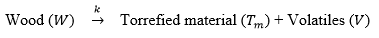The one-step reaction process can be presented as two reactions in parallel: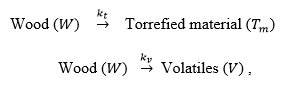where k is the overall reaction rate constant (min-1), kt is the reaction rate constant for the torrefied material (min-1), and kv is the reaction rate constant for the volatiles (min-1).

The elementary reaction rates for the decomposition of solid wood () and the torrefied material () over time can be presented using Eqs. (1) and (2), respectively.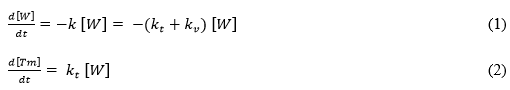The total solids after torrefaction () in the system can be determined using Eq. (3).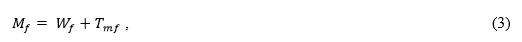where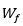(unreacted wood after the torrefaction process) and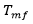(torrefied wood) are obtained from the integration of Eqs. (1) and (2).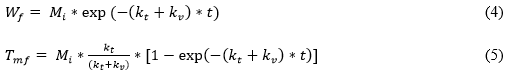Here, Mi is the sample mass before torrefaction (g) and t is the reaction time (min).

The fractions of wood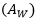and torrefied material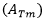after torrefaction, with respect to the sample mass before torrefaction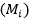can be written as: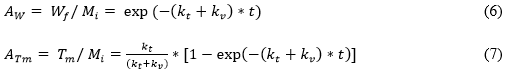Therefore, the fraction of total solids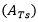in the system after torrefaction, with respect to the sample mass before torrefaction, is the sum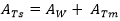.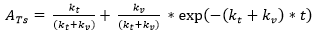(8)

When torrefaction time is sufficiently long, the fraction of total solids is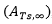, and Eq. (8) can be written as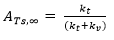(9)

Then, Eq. (8) becomes,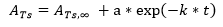(10)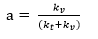(11)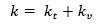(12)

where a is a dimensionless kinetic parameter for a first order reaction and k, the reaction rate constant, can be represented by the Arrhenius equation (Eq. 13). This equation shows the dependence of k in the chemical reaction on the absolute temperature (T (K)), the activation energy (Ea), the frequency or pre-exponential factor (k0), and R, the universal gas constant (8.314 Jmol-1K-1).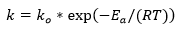(13)

Such a kinetics model will be able to predict the behavior of wood during the torrefaction process. That is, the kinetics model will be expected to describe the changing mass yield as a function of time and temperature. Likewise, this information will be basic for designing an optimized torrefaction unit.

A summary of the parameters used so far to determine the reaction rate constant for a torrefaction process, specifically the activation energy (Ea) and the pre-exponential factor (k0), are presented in Table 1. All data presented in Table 1 were obtained assuming a first-order reaction. An extra column has been added with the rate constant calculated at 300 °C. As mentioned before, the kinetics parameters’ results are a function of the raw material, reaction system, residence time, and temperature range. The pre-exponential factor shows the most widespread variation.

In the current research, a fluidized reactor was used to determine the torrefaction kinetics of red oak at temperatures between 230 and 330 °C.

Table 1. First-Order Kinetics Data from the Literature and Calculated Rate Constants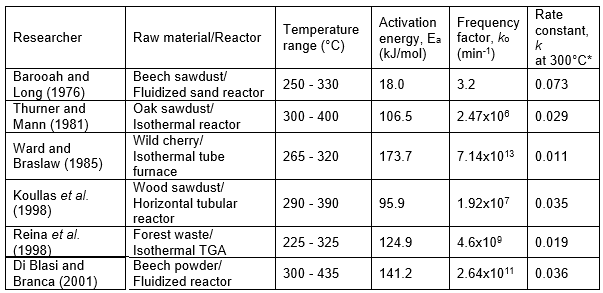Determined using the Arrhenius equation. Note that 300 °C = 573.15 K.

EXPERIMENTAL

Materials

Red oak was collected as wood chips (without bark) from a West Virginia sawmill. The raw material was first conditioned to 5% moisture content and ground into flour using a Wiley mill outfitted with a 1-mm mesh sieve. The flour wood was screened to the size of 0.5 to 0.71 mm, using sieves (25 to 35 U.S. mesh). The moisture content of all samples before the torrefaction process was 5.15% ± 0.03.

Torrefaction Reactor

A stainless steel fluidized reactor, along with a heating and cooling system, was designed and built at a laboratory level (Fig. 1). The reactor dimensions corresponded to a 300-mm length and a 50-mm diameter with an internal basket. The basket (125 cm3 and external diameter of 49.90 mm) was built using a perforated sheet of stainless steel (140 U.S. mesh); it was used to avoid losses of the torrefied material through the system. Approximately 25% of the basket volume contained the sample, and the rest was used as a fluidized zone. Ten grams of sample were used in each experiment, and nitrogen was flowed as an inert gas (35 L/min). Four reaction temperatures (230 °C, 270 °C, 300 °C, and 330 °C) and three residence times (10 min, 20 min, and 30 min) were used in the experiments (Table 2). The wood samples were introduced into the reactor when the system reached the desired torrefaction temperature. After torrefaction, the prevention of autoignition of the torrefied biomass was achieved through the use of an improved cooling system consisting of an indirect cold water coil used to open V-1 and direct fresh nitrogen used to open V-3 (Fig. 1). A temperature controller held the torrefaction temperature constant. The emerging torrefaction gases were cleaned in a scrubber unit before being vented into the hood.

Nineteen samples of red oak were prepared for the torrefaction experiments, and the details of these experiments are presented in Table 2.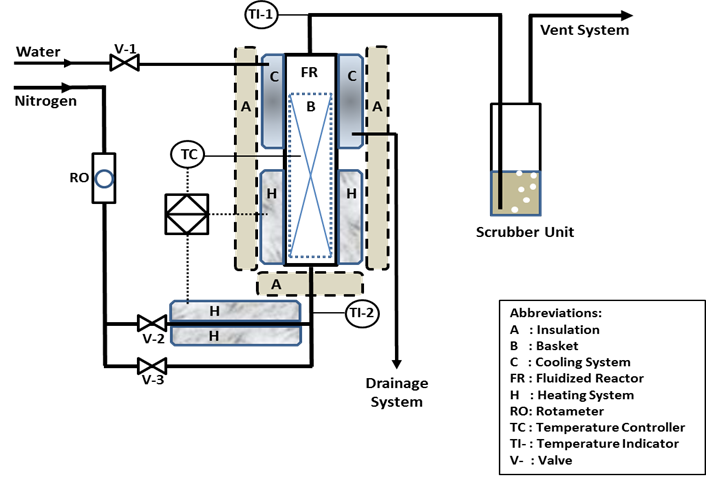Fig. 1. Fluidized reactor system

Table 2. Nomenclature and Parameters of Torrefied Red Oak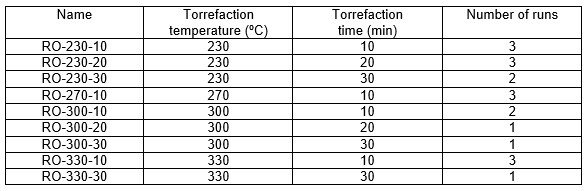Mass Loss

The mass loss (ML) of the samples after torrefaction was calculated using the following formula,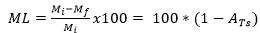, (14)

where Mi is the sample mass before torrefaction (g) and Mf is the sample mass after torrefaction (g).

Proximate Analysis

Proximate analysis of untreated and torrefied red oak was performed according to ASTM D3172-07. A LECO 701 proximate analyzer was used. The amount of material required corresponded to approximately 2 g. The results include the determination of moisture, volatile matter, ash, and fixed carbon.

Ultimate Analysis

Ultimate analysis of untreated and torrefied red oak was performed using a ThermoQuest Elemental Analyzer, FLASH EA 1112 series, according to ASTM D3176. The amount of sample required consisted of 1 to 2.5 mg. The elemental composition corresponds to nitrogen, carbon, sulfur, hydrogen, and oxygen. The percentage of oxygen was determined as the difference. The results corresponded to the average of three measurements and before and after each set of measurements a standard sample was analyzed to verify the performance of the equipment.

Kinetics

The mass loss (ML) of wood after the torrefaction experiment at a specific time and temperature was calculated according to Eq. (14). Using this mass loss, the parameter (Eq. (14)) was determined at different times and temperatures. The fraction of total solids at infinite time was determined from Eq. (10).

For different experimental times (t) with known parameters and , it was possible to obtain the values for a and k as functions of the torrefaction temperature through a graphic method using Eq. (10). Finally, using the natural logarithm of Eq. (10), it was possible to obtain a linear function with intercept ln(a) and slope k.

Considering that the wood samples were introduced into the hot reactor when the system reached the desired torrefaction temperature, it was assumed that at zero torrefaction time, the mass loss of the sample was zero. It was also assumed that the heat-up time for the sample was negligible.

By plotting Eq. (13) with different reaction rate constants as a function of the torrefaction temperature, it was possible to determine the activation energy (Ea) and the pre-exponential factor (k0).

RESULTS AND DISCUSSION

Mass Loss of Torrefied Red Oak

The change in the torrefaction temperature from 230 to 330 °C had a much more significant effect on the mass loss of the red oak than that seen for the change in the residence time (from 10 min to 30 min). For example, when the torrefaction time was raised from 10 to 30 min at 230 °C, the mass loss increased from 19.0% to only 23.2%, with a difference of 4.2% (according to Table 3). On the other hand, for a constant residence time of 10 min, an increase in temperature from 230 to 270 °C resulted in a change of mass loss from 19.0% to 39.6%, a difference of 20.6%.

Table 3. Mass Loss of Solid Product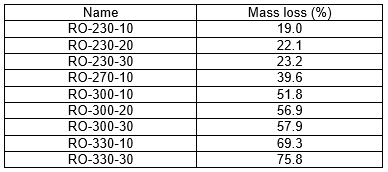The significant influence of the torrefaction temperature shown in Table 3 is similar to that reported by Bridgeman et al. (2010) and Medic et al. (2012) in earlier studies. Bridgeman et al.(2010) employed a horizontal tube furnace with willow (Salix spp.) and Chinese silvergrass (Miscanthus sinensis) as raw materials. They performed their torrefaction experiments in the temperature range between 240 and 290 °C and with residence times between 10 and 60 min. Also, they investigated the particle size of the raw material in the range of 4 to 20 mm. Bridgeman et al. (2010) concluded that torrefaction temperature is the most important parameter in terms of mass loss, compared with the residence time and the particle size. Likewise, Medic et al. (2012) concluded that the torrefaction temperature and raw biomass moisture content are the most important parameters during the torrefaction process. These authors used a fixed-bed reactor with corn stover as the biomass, in the temperature range of 200 and 300 °C and with residence times between 10 and 30 min. The moisture content of the raw feed varied between 3% and 41%.

Figure 2 shows the mass loss for red oak when the torrefaction temperature was increased from 230 to 330 °C in the present study. These data suggest that the mass loss is near to zero at approximately 200 °C: that is, the torrefaction process in our fluidized reactor begins at around 200 °C. This result is consistent with the information presented by others (Prins et al. 2006a; Peng et al. 2012; Bergman et al. 2005).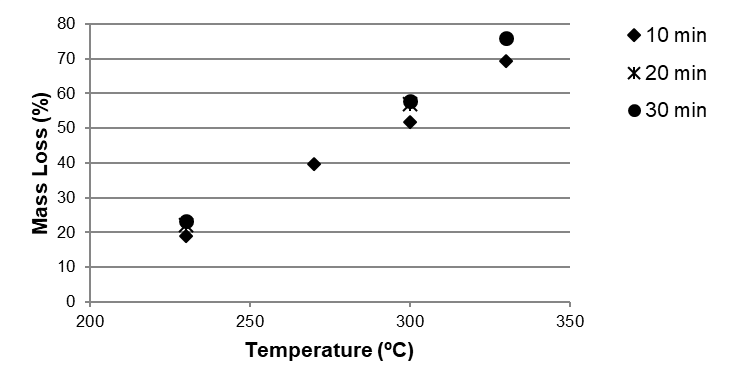Fig. 2. Mass loss of red oak at different temperatures and residence times

Gross Calorific Value

The resultant gross calorific values of the products after the torrefaction process are presented in Table 4. The data are presented only for the experiments performed at a residence time of 10 min. A significant increase in calorific value is noticeable when the temperature increases from 300 to 330 °C. However, the mass loss of nearly 70% obtained at 330 °C makes the torrefaction process at that temperature economically impracticable. Mass losses between 30 and 40% are traditionally regarded as more acceptable. The run at 270 °C with the 10-min residence time meets this requirement, with a mass loss of nearly 40%, and the product has a calorific value of just over 21,200 kJ/kg.

Table 4. Gross Calorific Value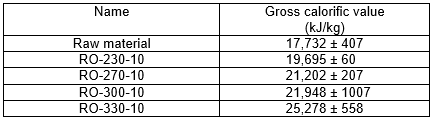In a fluidized reactor using samples of spruce and fir sawdust, Li et al. (2012) determined that a gross calorific value of 22,800 MJ/kg for torrefied material was obtained when the torrefaction temperature was 290 °C for 60 min with a mass loss of roughly 42%. The raw material presented a gross calorific value of 19,000 MJ/kg. Almeida et al. (2010) used an oven at 280 °C for 1 h and presented a calorific value of 21,800 MJ/kg and a mass loss of around of 23%. The raw material was Eucalyptus saligna, and it had a gross calorific value of 18,600 MJ/kg. Bridgeman et al. (2008) published a calorific value of 22,600 MJ/kg with a mass loss of roughly 38% (starting with a calorific value 18,900 MJ/kg for the raw material) at 294 °C and 45 min, using a TGA with wheat straw as the raw material. In this research, at 270 °C and a torrefaction time of 10 min, the resulting calorific value was 21,200 MJ/kg, with a mass loss of 39.6%, the raw material having an energy content of 17,700 MJ/kg. Comparing the results shown in this paragraph, it is possible that an increment in the energetic content of the raw material after a torrefaction of around 20% can be influenced by different factors, such as experimental devices (TGA, oven, and fluidized reactor), raw materials (spruce, fir, Eucalyptus saligna, wheat straw, and red oak), and operating conditions (temperature and residence time).

Table 4 shows that the highest standard deviation for gross calorific value occurs at around 300 °C. Likewise, Fig. 3 shows that at the same temperature, there is an increasing standard deviation, especially regarding the mass loss; several authors comment that there are more intense chemical reactions around this temperature associated with the decomposition of hemicellulose, cellulose, and lignin (Aziz et al. 2012; Bates and Ghoniem 2012; Bridgeman et al. 2008; Prins et al. 2006a). According to Medic et al. (2012), a torrefaction temperature of around 300 °C yields an important amount of condensable materials compared with the yield of permanent gases; this fact might be evidence of more intensive decomposition of not only hemicellulose but also cellulose and lignin. At 300 °C, the production of heavier compounds responsible for tar formation was observed in the condensed phase as well (Medic et al. 2012). Aziz et al. (2012) and Ciolkosz and Wallace (2011) show in their results that at temperatures between 200 and 300 °C, hemicellulose decomposition is more important than cellulose and lignin decomposition, and torrefaction is dominated by the thermal breakdown of hemicellulose to a combination of gases, condensable liquids, and solid components. Bridgeman et al.(2008) suggested that cellulose has a slower decomposition process at temperatures around 250 °C, and the rate of thermal decomposition is more rapid above 300 °C. They also stated that the decomposition is proportional to the carbon content in the torrefied material.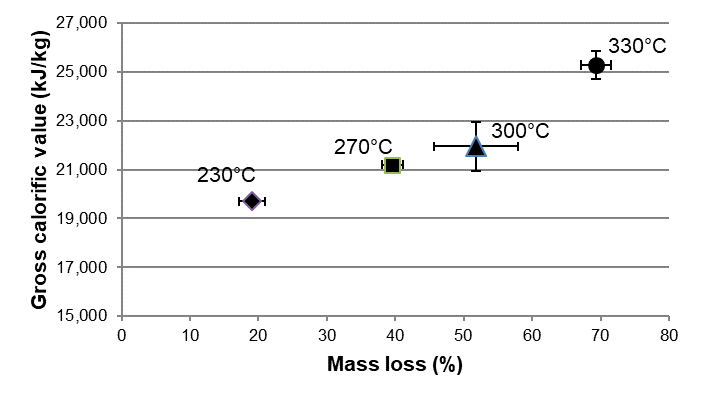Fig. 3. Gross calorific value as a function of mass loss after torrefaction

Proximate Analysis

Proximate analyses of the torrefied products are presented in Table 5. The final moisture content for the torrefied biomass was found to be nearly constant at around 3%, which is similar to the values presented by Bridgeman et al. (2010). The volatile matter underwent a strong change at around 300 °C; below 300 °C, the variation was around 10%, while above 300 °C, it was around 25%. The ash content for the red oak used here was extremely small and was lower than that presented by Prins et al. (2006b) using beech, willow, and straw. Moreover, there was an important increment in the fixed carbon at around 270 °C. When the temperature was below 270 °C (compared to ambient temperature), the change in the fixed carbon was roughly 35%, but for temperatures above 270 °C (until 330 °C), the variation in the fixed carbon was approximately 60%.

Table 5. Proximate Analyses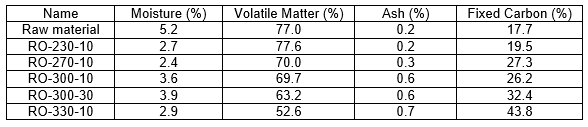Ultimate Analysis

Table 6 shows the elemental composition of red oak before and after the torrefaction process. No significant differences were noted between the raw material and the torrefied biomass in terms of the nitrogen and hydrogen compositions, with differences of only 0.5% and 2.0%, respectively. Sulfur was not detected. The most important changes after torrefaction with respect to untreated red oak were seen for carbon and oxygen, with differences of 19.3% and 17.5%, respectively. Below 300 °C, the incremental difference in elemental carbon was 6.7%, while above 300 °C, it rose to 12.6%.

Table 6. Elemental Composition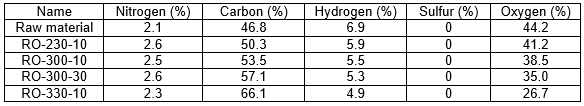The molar elemental ratios of the red oak before and after the torrefaction process are presented in Table 7.

Table 7. Molar Ratio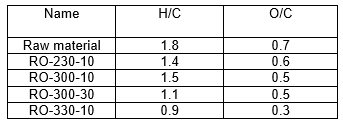H/C: Molar ratio between hydrogen and carbon

O/C: Molar ratio between oxygen and carbon

In general, the results correspond to those obtained by other authors (Bergman et al. 2005; Bridgeman et al. 2010; Phanphanich and Mani 2011). According to Kim et al. (2012) and Prins et al. (2006b), the energy contained in C-C bonds is higher than that in C-O and C-H bonds. Therefore, the incremental increase in C content and the reduction of the O/C and H/C ratios improve the calorific value in the torrefied material.

Kinetics Model for Torrefaction of Red Oak

The total solids fraction was used for the kinetics calculations using three temperatures (230, 300, and 330 °C). Figure 4 presents the experimental data for at different temperatures and residence times in our fluidized reactor. For the calculation of the total solid fraction at infinite time,, the value of at a 30-min residence time was selected (Eq. (10)). When the torrefaction temperature increased from 230 to 330 °C, the difference between changed from 0.043 to 0.065, from 10 min to 30 min, respectively. According to Fig. 4, is higher at a lower temperature; this result was expected, as the mass loss suggests a proportional behavior with respect to the torrefaction temperature. In other words, at a low torrefaction temperature, the mass loss is low and the fraction of total solids in the system after torrefaction is high.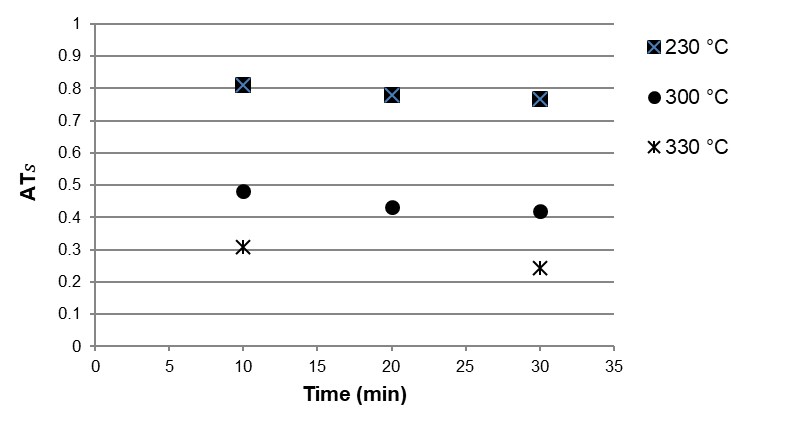Fig. 4. Total solids fraction as a function of time at different torrefaction temperatures

Noting that the reaction rate constant depends on the relationship between the fraction of total solids after torrefaction and the time for a desired torrefaction temperature, it is possible to rewrite Eq. (10) as: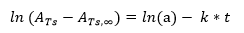(15)

The resulting plot of the data as shown in Fig. 5 suggests a linear relationship for the logarithm of the volatile mass fraction () vs. time at different torrefaction temperatures. This result means that for the fluidized reactor below the temperature range 230 to 330 °C and using red oak, the reaction rate coefficient presents a behavior approximately constant with respect to the time that mainly depends on the torrefaction temperature. Therefore, it is possible to use the Arrhenius equation (Eq. 13).

Considering the curve slopesobtained at different temperatures (230 °C, 300 °C, and 330 °C), it is possible to plot (Fig. 6) the different reaction rate constants as a function of the torrefaction temperature.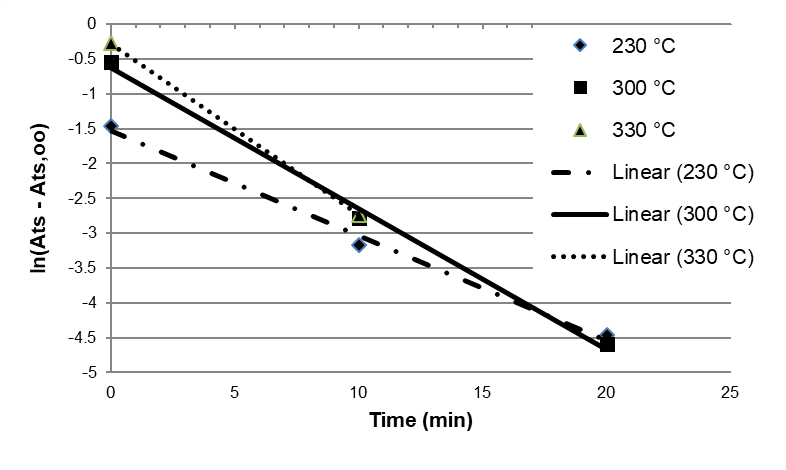Fig. 5. Logarithm of the volatile mass fraction versus time at different torrefaction temperatures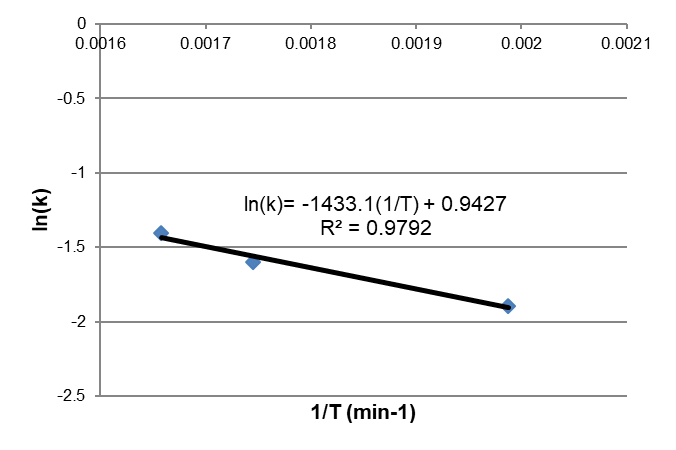Fig. 6. Parameters of the Arrhenius equation

The kinetic model obtained according to the methodology presented is the following,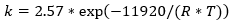(16)

where is the reaction rate constant (min-1), is the universal gas constant equal to 8.314 (J mol-1K-1), and is the temperature (K). The pre-exponential or frequency factor for the torrefaction process is 2.57 min-1, and the activation energy is 11.92 kJ/mol.

Figure 7 presents a summary of the reaction rate constants determined using Eq. (13) at temperatures of 230 °C (503.15 K), 270 °C (543.15 K), 300 °C (573.15 K), and 330 °C (603.15 K) for the kinetics data published by others (Table 1) plus the data generated in this work. The reaction rate constant determined in this study is the highest compared with the data presented in the literature for a first-order reaction, one-step mechanism, and temperature range between 230 to 330 °C; for instance, is 0.212 min-1 at 300 °C. The closest results with respect to the present study were obtained by Barooah and Long (1976) in a temperature range between 230 to 300 °C ( is 0.073 min-1 at 300 °C), and by Di Blasi and Branca (2001) only at the highest temperature of 330 °C ( is 0.036 min-1 at 300 °C). Both experiments were performed in a fluidized reactor. However, according to Table 1, the activation energy and frequency factor vary widely, from 18 to 173.7 kJ/mol and from 3.2 to 7.14×1013 min-1, respectively. These important variations may be caused by different heating conditions, raw materials with varied characteristics, various experimental devices, particular operating conditions, and use of different mathematical methods to fit the laboratory results.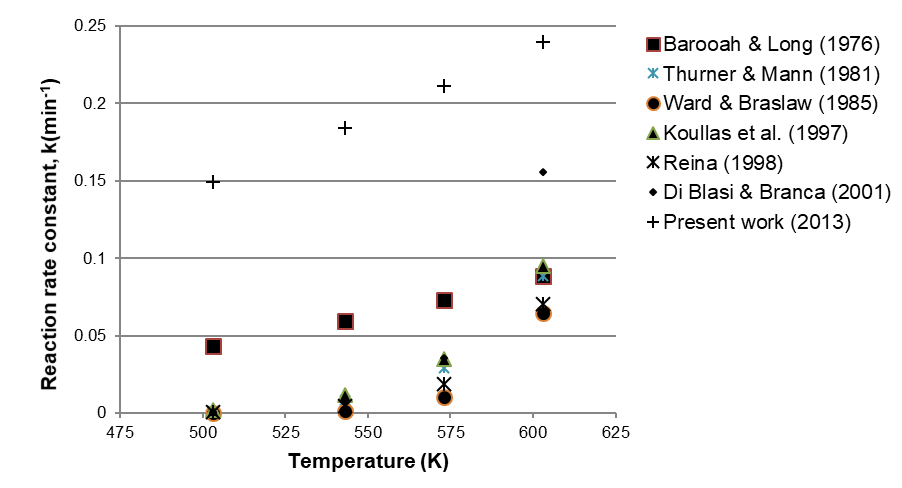Fig. 7. Values of reaction rate coefficients as a function of torrefaction temperature

Traditionally, torrefaction temperatures are between 200 and 300 °C; within this temperature range, the reaction rate coefficient obtained in this study is at least three times larger than the next closest one. This high reaction rate might be due to the fact that torrefaction in a fluidized reactor presents high mass and energy transfer coefficients; this is consistent with comments presented by Li et al. (2012), who mentioned that torrefaction of biomass in fluidized bed reactors offers improved heating efficiency.

Figure 8 shows the relationship, at different temperatures, between the fraction of total solids obtained after torrefaction in the fluidized reactor and the theoretical fraction of total solids obtained using Eq. (10). The obtained model (Eq. (10)) was evaluated at torrefaction temperatures of 230, 300, and 330 °C, and its maximum error was 1.33% at torrefaction temperatures of 330 °C and a residence time of 10 min.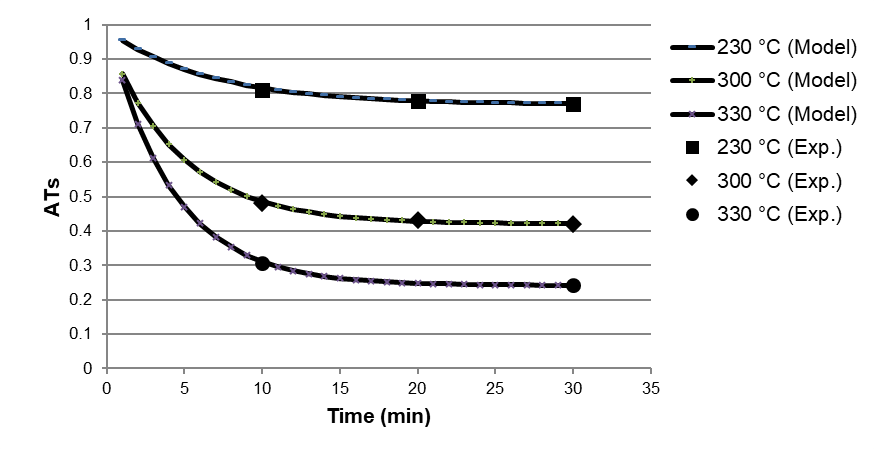Fig. 8. Fraction of total solids after torrefaction: a comparison of experimental and calculated results at torrefaction temperatures of 230 °C, 300 °C, and 330 °C

CONCLUSIONS

1. First-order kinetics and a one-step reaction can effectively predict the torrefaction kinetics of red oak in a fluidized reactor for temperatures ranging between 230 to 330 °C.
2. The reaction rate constant, k (min-1), for the torrefaction of red oak (Quercus rubra) using a bench-scale fluidized reactor is, k = 2.57*exp(-11920/(R*T)) where R is the universal gas constant 8.314 (J mol-1K-1) and T is the temperature (K). With this rate expression, one can determine the mass loss for red oak during torre-faction in a fluidized reactor in a temperature range between 230 and 330 °C.
3. The high value of the reaction rate constant generated using a fluidized system indicates that it is an excellent alternative for carrying out torrefaction. The torrefaction process will require less reaction time to generate a material with the desired energetic properties. However, there are different factors that can influence its value, such as raw materials, heating conditions, operating conditions, experimental devices, and mathematical methods used to fit the laboratory results.
4. The oxygen and hydrogen contents of the torrefied material decreased from 44.2% to 26.7% and 6.9% to 4.9%, respectively, when the torrefaction temperature and time increased, while the carbon content increased from 46.8% to 66.1%. A proportional relationship between energy content and weight loss was found for red oak: when the mass loss increased roughly from 19% to 69%, the energy content increased from approximately 19,700 kJ/kg to 25,300 kJ/kg. However, at 300 °C, an increase in the standard deviation of the data was found, mainly due to the onset of more intense chemical reactions associated with the decomposition of hemicellulose, cellulose, and lignin at this temperature.

ACKNOWLEDGMENTS

This research was funded by the WVU-Energy Research Grant (ERG) Program. ERG-Oporto, Award NT10042R- Project 10013855. Special thanks to Dr. David DeVallance, Assistant Professor of Wood Science and Technology – Division of Forestry and Natural Resources – West Virginia University, for his help with setting up the torrefaction system.

REFERENCES CITED

Almeida, G., Brito, J. O., and Perré, P. (2010). “Alterations in energy properties of eucalyptus wood and bark subjected to torrefaction: The potential of mass loss as a synthetic indicator,” Bioresource Technol. 101(24), 9778-9784.

Arias, B., Pevida, C., Fermoso, J., Plaza, M. G., Rubiera, F., and Pis, J. J. (2008). “Influence of torrefaction on the grindability and reactivity of woody biomass,” Fuel Process Technol.89(2), 169-75.

ASTM D2015: Standard Test Method for Gross Calorific Value of Coal and Coke by the Adiabatic Bomb Calorimeter.

ASTM D3172-07: Standard Practice for Proximate Analysis of Coal and Coke.

ASTM D3176: Standard Practice for Ultimate Analysis of Coal and Coke.

Aziz, M. A., Sabil, K. M., Uemura, Y., and Ismail, L. (2012). “A study on torrefaction of oil palm biomass,” J. Applied Sci. 12(11), 1130-1135.

Barooah, J. N., and Long, V. D. (1976). “Rates of thermal decomposition of some carbonaceous materials in a fluidized bed,” Fuel 55(2), 116-120.

Bates, R. B., and Ghoniem, A. F. (2012). “Biomass torrefaction: Modeling of volatile and solid product evolution kinetics,” Bioresource Technol. 124(11), 460-469.

Bergman, P. C. A., and Kiel, J. H. A. (2005). “Torrefaction for biomass upgrading,” Energy Research Centre of the Netherlands (ECN) Publication No ECN-RX-05-180. (http://www.ecn.nl/docs/library/report/2005/rx05180.pdf).

Bergman, P. C. A., Boersma, A. R., Kiel, J. H. A., Prins, M. J., Ptasinski, K. J., and Janssen, F. J. J. G. (2005). “Torrefaction for entrained – flow gasification of biomass,” Energy Research Centre of the Netherlands (ECN) Publication No ECN-C-05-067. (ftp://nrg-nl.com/pub/www/library/report /2005/c05067.pdf).

Bridgeman, T. G., Jones, J. M., Shield, I., and Williams, P. T. (2008). “Torrefaction of reed canary grass, wheat straw and willow to enhance solid fuel qualities and combustion properties,” Fuel 87(6), 844-856.

Bridgeman, T. G., Jones, J. M., Williams, A., and Waldron, D. J. (2010). “An investigation of the grindability of two torrefied energy crops,” Fuel 89(12), 3911-3918.

Chen, W. H., and Kuo, P. C. (2011). “Isothermal torrefaction kinetics of hemicellulose, cellulose, lignin and xylan using thermogravimetric analysis,” Energy 36(11), 6451-6460.

Ciolkosz, D., and Wallace, R. (2011). “A review of torrefaction for bioenergy feedstock production,” Biofuel. Bioprod. Bior. 5(3) 317-329.

Deng, J., Wang, G. J., Kuang, J. H., Zhang, Y. L., and Luo, Y. H. (2009). “Pretreatment of agricultural residues for co-gasification via torrefaction,” J. Anal. Appl. Pyrol. 86(2), 331-337.

Di Blasi, C., and Branca, C. (2001). “Kinetics of primary product formation from wood pyrolysis,” Ind. Eng. Chem. Res. 40(23), 5547-5556.

Fisher, E. M., Dupont, C., Darvell, L. I., Commandre, J. M., Saddawi, A., Jones, J. M., Grateau, M., Nocquet, T., and Salvador, S. (2012). “Combustion and gasification characteristics of chars from raw and torrefied biomass,” Bioresource Technol. 119(9), 157-165.

Kim, Y. H., Lee, S. M., Lee, H. W., and Lee, J. W. (2012). “Physical and chemical characteristics of products from the torrefaction of yellow poplar (Liriodendron tulipifera),” Bioresource Technol. 116(6), 120-125.

Kleinschmidt, C. (2011). “Overview of international developments in torrefaction”. Central European Biomass Conference. Graz, Austria. (http://www.bioenergytrade.org /pastevents/graz.html).

Koullas, D. P., Nikolaou, N., and Koukios, E. G. (1998). “Modeling non-isothermal kinetics of biomass prepyrolysis at low pressure,” Bioresource Technol. 63(3), 261-266.

Li, H., Liu, X., Legros, R., Bi, X. T. T., Lim, C. J., and Sokhansanj, S. (2012). “Torrefaction of sawdust in a fluidized bed reactor,” Bioresource Technol. 103(1), 453-458.

Medic, D., Darr, M., Shah, A., Potter, B., and Zimmerman, J. (2012). “Effects of torrefaction process parameters on biomass feedstock upgrading,” Fuel 91(1), 147-154.

Oliveira, T., and Rousset, P. L. A. (2009). “Effects of torrefaction on energy properties of Eucalyptus grandis wood,” Cerne 15(4), 446-452.

Peng, J. H., Bi, X. T. T., Lim, J., and Sokhansanj, S. (2012). “Development of torrefaction kinetics for British Columbia softwoods,” Int. J. Chem. Reactor Eng. 10(1), 1-37.

Phanphanich, M., and Mani, S. (2011). “Impact of torrefaction on the grindability and fuel characteristics of forest biomass,” Bioresource Technol. 102(2), 1246-1253.

Prins, M., Ptasinski, K. J., and Janssen, F. J. J. G. (2006a). “Torrefaction of wood. Part 1: Weight loss kinetics,” J. Anal. Appl. Pyrol. 77(1), 28-34.

Prins, M., Ptasinski, K. J., and Janssen, F. J. J. G. (2006b). “Torrefaction of wood. Part 2: Analysis of products,” J. Anal. Appl. Pyrol. 77(1), 35-40.

Reina, J., Velo, E., and Puigjaner, L. (1998). “Kinetic study of the pyrolysis of waste wood,” Ind. Eng. Chem. Res. 37(11), 4290-4295.

Thurner, I., and Mann, U. (1981). “Kinetic investigation of wood pyrolysis,” Ind. Eng. Chem. Process. Des. Dev. 20(3), 482-488.

Ward, S. M., and Braslaw, J. (1985). “Experimental weight loss kinetics of wood pyrolysis under vacuum,” Combust. Flame 61(3), 261-269.

Zheng, A., Zhao, Z., Chang, S., Huang, Z., He, F., and Li, H. (2012). “Effect of torrefaction temperature on product distribution from two-staged pyrolysis of biomass,” Energ. Fuel 26(5), 2968-2974.

Article submitted: June 17, 2013; Peer review completed: August 5, 2013; Revised version received and accepted: August 13, 2013; Published: August 16, 2013.